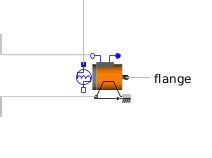Time Table - MapleSim Help

Time Table

Generate output from a time-based lookup tableDescription The Time Lookup Table (or Time Table) component generates an output signal by interpolation using time as the input. The input values of the data table (that is, the first column of values) must be increasing; however, a discontinuous function can be created by repeating an input value. The start time parameter is subtracted from the actual simulation time and the resulting time is used as the interpolation time. The offset parameter is added to the computed interpolation value. The interpolation value is 0 if the interpolation time is less than the first time point.Data Source Options

The data source parameter selects the source for the data. It can be either file, attachment, or inline.

 • file: the data is saved in an Excel or a CSV file on the hard drive. Use the data parameter to browse to and select the data file.
 • attachment: the data set is attached to the MapleSim model. Use the data parameter to select the attachment that contains the data set.
 Note: In the Attachments pane, your data set file must be attached in the Data Sets category. For more information, see Attaching a File to a Model.
 – Attach a Microsoft® Excel® (.xls/.xlsx) or comma separated value (.csv) file containing the data values to the model.
 – Generate a data set in the Apps Manager tab using either the Data Generation app or Random Data Generation app. Data sets that you generate have the .csv file extension. For more information about MapleSim apps, see Opening MapleSim Apps and Templates.
 • inline: enter the data table in the table parameter as an m (rows) by n (columns) matrix.
 – To change the dimensions of table, right-click (Control-click for Mac®) the parameter field and select Edit Matrix Dimensions. In the Matrix Dimensions dialog, enter values for the number or rows and columns and then click OK.

For all data source options, the first column in the data table represents the time. The other columns, $2$ through n, represent the output data.

The columns parameter selects which data column to output if your data table has more than two columns. By default, the second column, $\left[2\right]$, is output.

You can output an array by entering an array in columns. For example, set columns to $\left[2,3,4\right]$ to output a three-element array corresponding to the data in the second, third, and fourth columns of your data table.Connections

 Name Description Modelica ID $y$ Real output of dimension $n$, the length of the $\mathrm{columns}$ parameter yParameters

 Name Default Units Description Modelica ID $\mathrm{data source}$ $\mathrm{inline}$ Specifies data source; see section (above) datasourcemode $\mathrm{table}$ $\left[\begin{array}{cc}0& 1\end{array}\right]$ Data set table $\mathrm{file name}$ $\mathrm{DataFileName}$ The path to the external file fileName $\mathrm{data}$ Attached data set data $\mathrm{columns}$ $\left[2\right]$ Data columns used.  In a spreadsheet, column A corresponds to 1. columns $\mathrm{offset}$ $0$ Offset of output signal $y$ offset $\mathrm{start time}$ $0$ $s$ Output y = offset for time < startTime startTime $\mathrm{skip rows}$ $0$ Number of header rows to skip skiprows $\mathrm{smoothness}$ Linear interpolation Selects interpolation: linear, cubic spline, or none smoothnessModelica Standard Library The component described in this topic is from the Modelica Standard Library. To view the original documentation, which includes author and copyright information, click here.